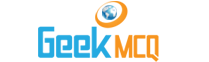# Engineering :: Refrigeration and Air conditioning

51.  If the suction pressure of a refrigeration compressor is too high, it shows
 A. Lack of refrigerant on cycle B. Excessive load and evaporator C. Negligible load on evaporator D. Any of above E. None of the above

52.  If a one ton refrigeration machine, the significance of term "One ton" is that
 A. One ton of refrigerant is used B. One ton is the total weight of equipment C. One ton water can be stored at a time D. One ton of water can be converted into ice E. One ton of ice when melts from and at 0?C in 24 hours the refrigeration effect equivalent to that

53.  A Bell-Coleman cycle is
 A. Reversed Carnot cycle B. Reversed Joule cycle C. Reversed Otto cycle D. Reversed Rankine cycle E. Reversed Sterling cycle

54.  In a refrigerating system work done per kg of air is 20 kcal and heat extracted per kg of air is 25 kcal. The quantity of refrigerant used is 10 kg. The coefficient of performance of the system would be
 A. 0.8 B. 8 C. 1.25 D. 12.5 E. 5

55.  In a vapour compression cycle the vapour as it leaves the compressor is
 A. In liquid form B. Wet vapour C. Dry vapour D. Dry and saturated vapour E. None of the above

56.  In a vapour compression cycle the refrigerant immediately after expansion valve is in
 A. Liquid form B. Wet vapour form C. Dry vapour form D. Dry and saturated vapour form E. None of the above

57.  The ability of an evaporator to absorb heat does not depends on
 A. Its size, surface condition and material B. Kind of refrigerant used C. Temperature difference between the evaporator and the surrounding air D. Velocity of turbulence E. Compressor speed

58.  A condenser removes from the refrigerant
 A. Sensible heat only B. Latent heat only C. Sensile heat and latent heat D. Partly sensible heat and latent heat E. None of the above

59.  A critical temperature is the temperature
 A. Below which gas is always liquefied B. Above which a gas will never be liquefied C. Below which a gas does not obey gas laws D. Above which a gas may explode E. None of the above

60.  The critical pressure of a liquid is the pressure
 A. Below which a liquid will always be in vapour form B. Above which a liquid will remain a liquid C. Above which a liquid will always turn into a vapour D. Above which a liquid will explode E. None of the above